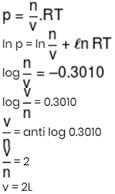Courses

# JEE Main Chemistry Mock - 2

## 25 Questions MCQ Test Mock Test Series for JEE Main & Advanced 2021 | JEE Main Chemistry Mock - 2

Description
This mock test of JEE Main Chemistry Mock - 2 for JEE helps you for every JEE entrance exam. This contains 25 Multiple Choice Questions for JEE JEE Main Chemistry Mock - 2 (mcq) to study with solutions a complete question bank. The solved questions answers in this JEE Main Chemistry Mock - 2 quiz give you a good mix of easy questions and tough questions. JEE students definitely take this JEE Main Chemistry Mock - 2 exercise for a better result in the exam. You can find other JEE Main Chemistry Mock - 2 extra questions, long questions & short questions for JEE on EduRev as well by searching above.
QUESTION: 1

### The compound 'A' when treated with methyl alcohol and few drops of H₂SO₄ give wintergreen smell. The compound 'A' is

Solution:

Salicylic acid gives wintergreen smell with methyl alcohol and H2SOto form Methyl salicylate.QUESTION: 2

### Which of the following will not give iodoform test?

Solution:

Benzyl alcohol does not have the CH3CO- group or CH3CH2Oso it will not give the positive iodoform test.

QUESTION: 3

### Phospholipids are esters of glycerol with

Solution:

Phospholipids are esters of glycerol with two carboxylic acid residue and one phosphate group. Hence, phospholipids may be regarded as derivative of glycerol in which two hydroxyl group are esterified with a fatty acid, while third is esterified with phosphoric acid.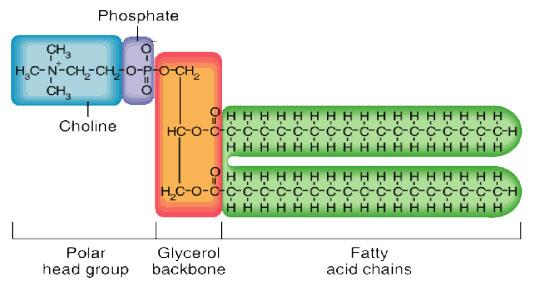QUESTION: 4

In graphite, the electrons are

Solution:

Carbon has four spare electrons. Electron that forms pi bond is freer to travel between atoms compared to the others and is theoretically associated with all adjacent atoms (imagine the 3d structure of graphite) rather than a single atom. Because of that, it is called delocalized electron.

QUESTION: 5

The maximum number of unpaired d-electrons are in

Solution:

Fe+3 has 5 unpaired electrons, more than other ones.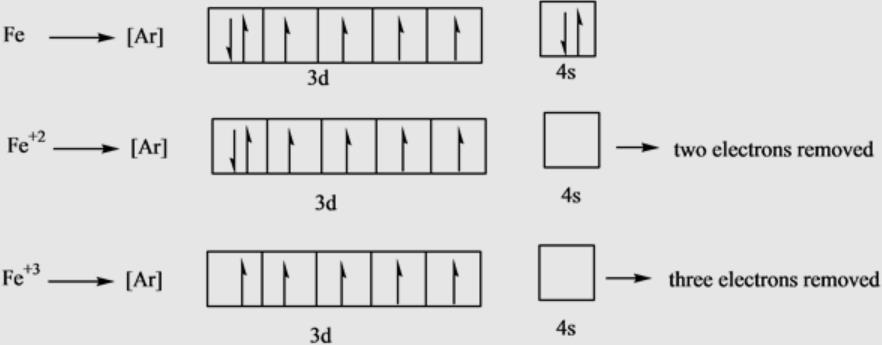QUESTION: 6

Splitting of spectral lines under the influence of magnetic field is called

Solution:
• Splitting of spectral lines under the influence of magnetic field is called Zeeman effect.
• In stark effect spectral lines split due to electricity.
• In photoelectric effect the splitting of spectral line takes place as the light falls on the metal.
QUESTION: 7

Alkyl groups are o- and p-directing because of

Solution:

Hyperconjugation effect in Alkyl group: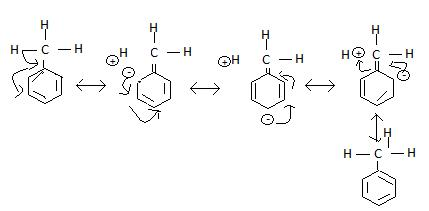QUESTION: 8

In which of the following molecule, all the atoms lie in one plane?

Solution:

It has got sp2 hybridization, giving it a planar structure.Further backbonding from flouine makes the B-F bonds stronger than normal single bonds.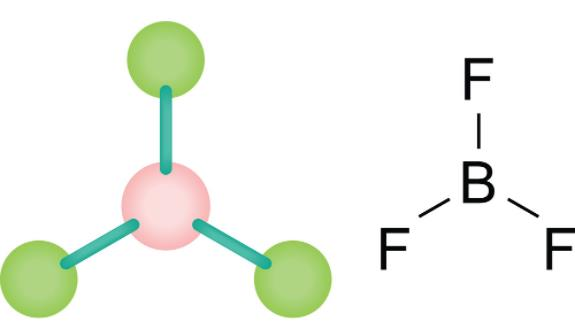QUESTION: 9

Pairs of species having identical shapes for molecules is

Solution:
• XeF2 contains sp3d hybridized Xe atom with three lone pairs of electrons.
• Fluorine atoms occupy axial positions while three lone pairs of electrons occupy equatorial positions in trigonal bipyramid.
• Since the lone pair of electrons does not consider for the shape of the molecule, thus, the shape of XeF2 comes out to be linear.
• While C -atom of CO2 is sp hybridised and thus, possesses a linear shape.QUESTION: 10

Which one of the following is expected to exhibit optical isomerism

Solution:

Optical isomers of cis-[Co(en)₂Cl₂] :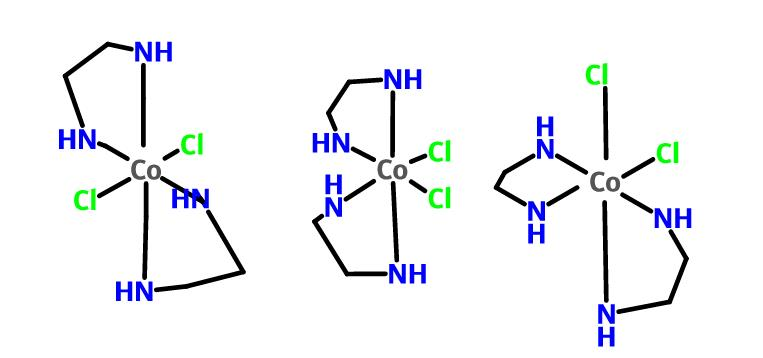QUESTION: 11

Nickel is purified by

Solution:

The Mond process, sometimes known as the carbonyl process, is a technique created by Ludwig Mond in 1890, to extract and purify nickel. The process was used commercially before the end of the 19th century. This process converts nickel oxides into pure nickel.

QUESTION: 12

The oxidation state of Cr in [Cr(NH₃)₄Cl₂]⁺ is

Solution:

Applying sum of individual charge = charge on molecule
x+(0)×4 + (-1)×2 = +1
x=3

QUESTION: 13

Which of the following noble gas is least polarisable?

Solution:

Helium is the least polarizable noble gas due to presence of weakest Van der Waal's forces.

QUESTION: 14

The IUPAC name of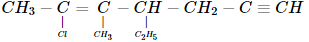Solution:

Correct name is 2-Chloro-4-ethyl-3-methyl hept-2-ene-6-yne

QUESTION: 15

When HCl gas is passed through a saturated solution of common salt, pure NaCl is precipitated because

Solution:

When hydrogen chloride gas (HCl) is circulated through the saturated solution.
HCl and NaCl dissociate into their respective ions as:

NaCl → Na+ Cl-

HCl → H+ + Cl-

The concentration of Cl− ions increases considerably in solution due to ionization HCl.

Hence, the ionic product [Na+][Cl−] exceeds the solubility product of sodium chloride and, therefore, pure sodium chloride precipitates out from the solution.

QUESTION: 16

Which of the following pair represents stereoisomerism?

Solution:
• Optical isomerism (such as enantiomers and diastereoisomers) and geometric isomerism (cis trans isomerism) represents stereoisomerism.
• Stereoisomers have the same molecular formula and same connectivity of atoms, but differ in the orientations of atoms.
• Chain isomerism, position isomerism and functional group isomerism represents structural isomerism.
QUESTION: 17

Which of the following pair of elements belongs to same period of the periodic table?

Solution:

Calcium and zinc both belong to the same period, i.e Period 4

QUESTION: 18

Natural rubber is a polymer of

Solution:

Natural rubber is from the monomer cis-isoprene (2-methyl-1,3-butadiene).
It is an addition polymer that is obtained as a milky white fluid known as latex from a tropical rubber tree.

QUESTION: 19

In view of their low ionization energies, the alkali metals are

Solution:

Alkali metals can readily lose their lone electron to form +1 oxidation state and can easily ionize this electron and this ns1 configuration results in alkali metals to have large ionic and atomic radii and they have high second ionization energy. This ionization energy decreases down the group and act as strong reducing agent.

QUESTION: 20

100 ml of a liquid A was mixed with 25 ml of a liquid B to give B to give a non-ideal solution of A-B mixture. The volume of this mixture would be

Solution:

For non-ideal solution, ΔVmix ​< 0.

Final volume decreases due to negative deviation from original volume 125 ml.
Hence, total volume will be little less than (100 + 25 = 1.25 mL).

*Answer can only contain numeric values
QUESTION: 21

Total number of species in which atleast one atom have same hybridization as in central atom of azide ion.
N2O, C2H2, CO2, C3O2, BeF2, NO2, PF3

Solution:

N2O, C2H2, CO2, C3O2, BeF2

*Answer can only contain numeric values
QUESTION: 22

How many chiral centers are in the following compound?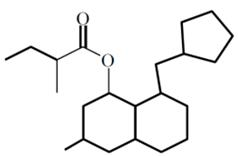Solution: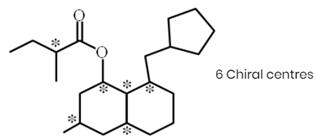*Answer can only contain numeric values
QUESTION: 23

An ideal solution was found to have a vapour pressure of 80 torr when the mole fraction of a non-volatile solute was 0.2. What would be the vapour pressure of the pure solvent at the same temperature?

Solution: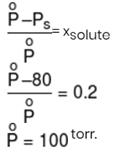*Answer can only contain numeric values
QUESTION: 24

What is the final temperature (in kelvin) of 0.10 mole monoatomic ideal gas that performs 75 cal of work adiabatically if the initial temperature is 227°C ? (use R = 2 cal/K-mol)

Solution: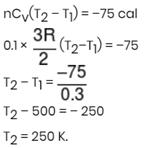*Answer can only contain numeric values
QUESTION: 25

log p v/s log RT curve plotted for 1 mole ideal gas. Calculate molar volume occupied by gas? (in liter)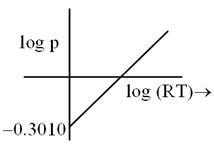Solution: